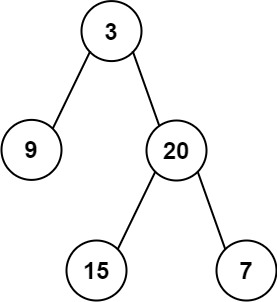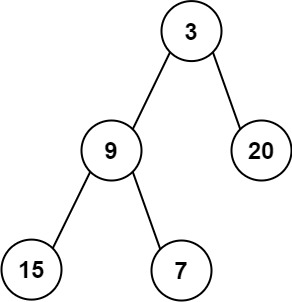# 637. Average of Levels in Binary Tree

Easy
Given the `root` of a binary tree, return the average value of the nodes on each level in the form of an array. Answers within `10-5` of the actual answer will be accepted.
Example 1:Input: root = [3,9,20,null,null,15,7]
Output:
[3.00000,14.50000,11.00000]
Explanation: The average value of nodes on level 0 is 3, on level 1 is 14.5, and on level 2 is 11.
Hence return [3, 14.5, 11].
Example 2:Input: root = [3,9,20,15,7]
Output:
[3.00000,14.50000,11.00000]
Constraints:
• The number of nodes in the tree is in the range `[1, 104]`.
• `-231 <= Node.val <= 231 - 1`

### 解題

Runtime: 12 ms, faster than 68.93%
Memory Usage: 6.7 MB, less than 54.12%
/**
* Definition for a binary tree node.
* type TreeNode struct {
* Val int
* Left *TreeNode
* Right *TreeNode
* }
*/
func averageOfLevels(root *TreeNode) []float64 {
nodeCount:= make(map[int]int)
value:= make(map[int]int)
maxIndex:= 0
var helper func(*TreeNode, int)
helper = func(root *TreeNode, index int) {
if root == nil {
return
}
nodeCount[index]++
value[index] += root.Val
if index > maxIndex { maxIndex = index }
helper(root.Left, index+1)
helper(root.Right, index+1)
}
helper(root, 0)
ans := make([]float64, 0)
for i:=0; i<=maxIndex; i++ {
ans = append(ans, float64(value[i]) / float64(nodeCount[i]))
}
return ans
}